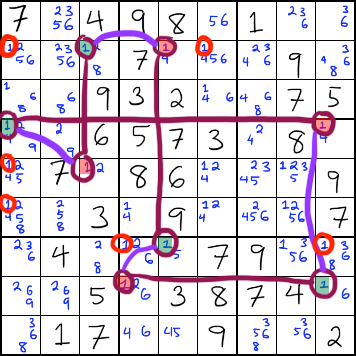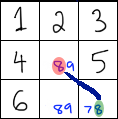## X-Cycles Strategy

X-Cycles is a strategy very similar to Simple Coloring. We deal with a single candidate and look for conjugate pairs, then we give each candidate alternating colors. However, the X-Cycles strategy can only be used when the chain forms a loop.

Contents

### When to use X-Cycles

When doing simple coloring, if you discover that the numbers are connected in a loop, you can use the X-Cycle strategy to see if any candidates can be eliminated.

### How to use X-Cycles

#### Even Number of Candidates in the Loop

If the loop has an even number of candidates, then we know that the loop itself has no flaws or contradictions within it. Either one color or the other is correct. For this reason, we can eliminate any candidates that can see two or more of the candidates in the loop.

(Play this Example)In the above image, there are eight 1s in the cycle: an even number. Because of the even number, we can eliminate all of the 1s that can see two or more candidates in the loop. The eliminated candidates in this example are circled in red.

If the loop has an odd number of candidates, we need to use the idea of weak links and strong links. Below, I explain what weak links and strong links are.

When there is a conjugate pair, the link between the two numbers in the pair can either be considered a weak link or a strong link. Below is the explanation of what each one is.

When two of the same candidate occur in the same unit together, they can be considered a conjugate pair. If that candidate occurs more than twice in that unit, then the link of the conjugate pair is considered a weak link.

When a conjugate pair has a weak link, you can say that if one of them is true, then the other is NOT true.In the above example, I show a link between two 8s in a box. These 8s share a WEAK link because there is another 8 in the box with the linked 8s.

If the candidate of a conjugate pair doesn't occur anywhere else in the unit except in the pair itself, then the link of the pair is considered to be a strong link.

When a conjugate pair has a strong link, you can say that if one of them is NOT true, then the other is true.In the above example, the 8s share a STRONG link because there are no other 8s in the same unit.

If there is an odd number of candidates in the loop, then we look for a candidate that has two strong links connected to it. If there is such a candidate, then we know that that candidate must be correct, and we can solve that square.

Why does this work? If two oppositely-colored candidates (let's call them A and B) have a strong link with a candidate (let's call it C), then you can say that if A or B is NOT true, then C MUST be true. But you know that only one of A or B can be true because they are opposite colors. One of them will definitely NOT be true. And because of that, C definitely MUST be true.

If there is an odd number of candidates in the loop, then we look for a candidate that has two weak links connected to it. If there is such a candidate, then we know that that candidate must be incorrect, and we can eliminate it.Why does this work? If two oppositely-colored candidates (let's call them D and E) have a weak link with a candidate (let's call it F), then you can say that if D or E IS true, then F must NOT be true. But you know that since D or E have opposite colors, one of them MUST be true. Because of that, F definitely CAN'T be true.